# College Math Foundations Flashcards

College Math Foundations Flashcards
1/32 (missed) 0 0
Create Your Account To Continue Studying

As a member, you'll also get unlimited access to over 79,000 lessons in math, English, science, history, and more. Plus, get practice tests, quizzes, and personalized coaching to help you succeed.

Try it risk-free for 30 days. Cancel anytime
Least common multiple of 6, 9 and 15
90
Got it
Least common multiple of 4, 7 and 8
56
Got it
Least common multiple of 6 and 10
30
Got it
Least common multiple of 4 and 5
20
Got it
Greatest common factor of 24 and 84
12
Got it
Greatest common factor of 9 and 27
9
Got it
Greatest common factor of 6, 24 and 33
3
Got it
Greatest common factor of 16 and 36
4
Got it
Greatest common factor of 10, 15 and 35
5
Got it
The distance between (-2,2) and (6,2)
8
Got it
The radius of a circle with center (0,5) and an endpoint on the circle (3,9)
5
Got it
The midpoint of a line with endpoints (1,-10) and (3,4)
(2,-3)
Got it
The midpoint of a line with endpoints (0,-2) and (2,4)
(1,1)
Got it
Center of a circle with diameter endpoints (-5,-2) and (3,-2)
(-1,-2)
Got it
The midpoint formula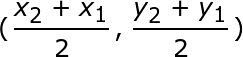Got it
The distance formula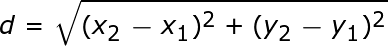Got it

or choose a specific lesson: See all lessons in this chapter
32 cards in set

## Flashcard Content Overview

The flashcards in this set cover a wide range of introductory math topics. There are flashcards that test definitions of terms, such as prime number and factor tree. Other cards test retention of key formulas, like the distance formula and the midpoint formula. Many cards in the set require you to put your knowledge to work. You'll practice finding the greatest common factor, least common multiple and the prime factors of various numbers. You'll also identify parts of graphs and symbols used in number sets.

Front
Back
The distance formulaThe midpoint formulaCenter of a circle with diameter endpoints (-5,-2) and (3,-2)
(-1,-2)
The midpoint of a line with endpoints (0,-2) and (2,4)
(1,1)
The midpoint of a line with endpoints (1,-10) and (3,4)
(2,-3)
The radius of a circle with center (0,5) and an endpoint on the circle (3,9)
5
The distance between (-2,2) and (6,2)
8
Greatest common factor of 10, 15 and 35
5
Greatest common factor of 16 and 36
4
Greatest common factor of 6, 24 and 33
3
Greatest common factor of 9 and 27
9
Greatest common factor of 24 and 84
12
Least common multiple of 4 and 5
20
Least common multiple of 6 and 10
30
Least common multiple of 4, 7 and 8
56
Least common multiple of 6, 9 and 15
90
A factor tree
A tool that diagrams how a number is split into its prime factors
Prime number
A number that can only be divided by 1 and itself
The prime factorization of 24
2 x 2 x 2 x 3
The prime factorization of 30
2 x 3 x 5
The prime factorization of 84
2 x 2 x 3 x 7
Identify the part of the graph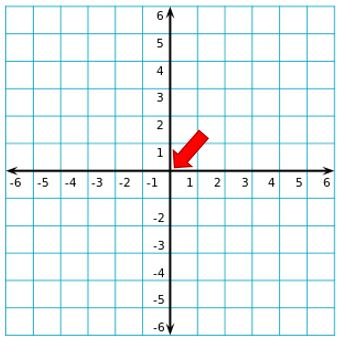Origin
Identify the part of the graph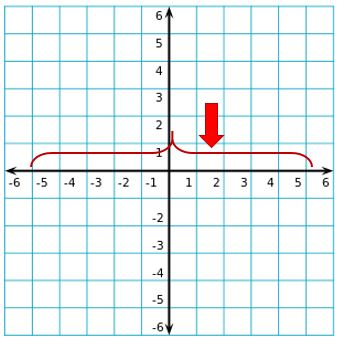x-axis
Identify the part of the graph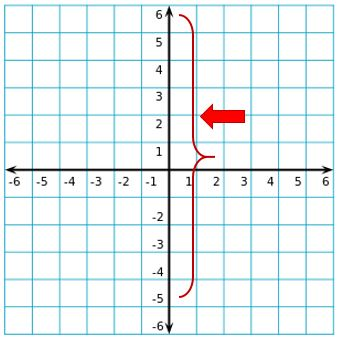y-axis
In the Venn diagram, the number of people who want both ice cream and cake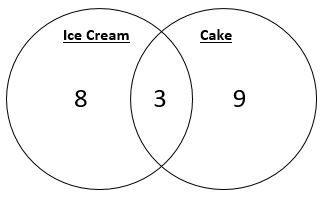3
The meaning of this symbol: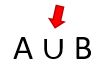This symbol means union. This example represents the union of sets A and B, which includes all of the elements from set A and set B.
The meaning of this symbol: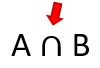This symbol means intersection. This example represents the intersection of sets A and B, which only includes the elements that are in both set A and set B.
Equivalent sets
Sets that contain the same number of elements
Identify the greatest common factor: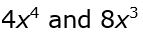The greatest common factor of 4 and 8 is 4. The greatest common factor of x4 and x3 is x3. So the greatest common factor is: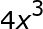Identify the greatest common factor: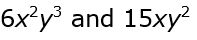The greatest common factor of 6 and 15 is 3. The greatest common factor of x2 and x is x. The greatest common factor of y3 and y2 is y2. So the greatest common factor is: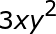Identify the least common multiple of 8y4 and 12y7

24y7

The least common multiple of 8 and 12 is 24. The least common multiple of y4 and y7 is y7.

Identify the least common multiple of 4x3y2 and 6x2y4

12x3y4

The least common multiple of 4 and 6 is 12. The least common multiple of x3 and x2 is x3. The least common multiple of y2 and y4 is y4.

To unlock this flashcard set you must be a Study.com Member.